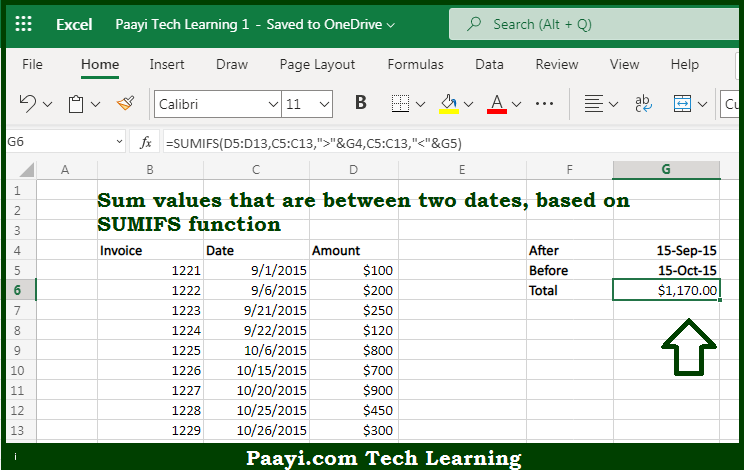# Learn How to SUM If Date is In Between in Microsoft Excel

Written by | 0 Comments | 352 Views

In this article, you will learn how to SUM various things in Microsoft Excel using a single/combination(s) of functions. You will also know how to SUM If Date is In Between and see the generic formula.

SUM If Date is In Between in Microsoft Excel

The main purpose of this formula is to sum values that are between two dates. Here we will learn how to sum the provided values between the dates in the workbook in Microsoft Excel. That implies, with the help of a formula based on the SUMIFs function you can able to sum values that are between two dates. So, with the help of this formula, you can able to sum the provided values between the dates in the workbook in Microsoft Excel.

General Formula to SUM If Date is In Between

=SUMIFS(rng1,rng2,">"&A1,rng2,"<"&B1)

The Explanation for the SUM If Date is In BetweenSo we know that with the help of the given formula above you can able to sum values that are between two dates. Here we will learn how to sum the provided values between the dates in the workbook in Microsoft Excel. As we know that the SUMIFS function allows logical operators like - "=",">",">=", etc., and can efficiently manage multiple criteria. To target values between two dates, we need to use two criteria. Each is entered as a criteria/range pair. So, with the help of this formula, you can able to sum if cells is having any specific text string in the workbook in Microsoft Excel.